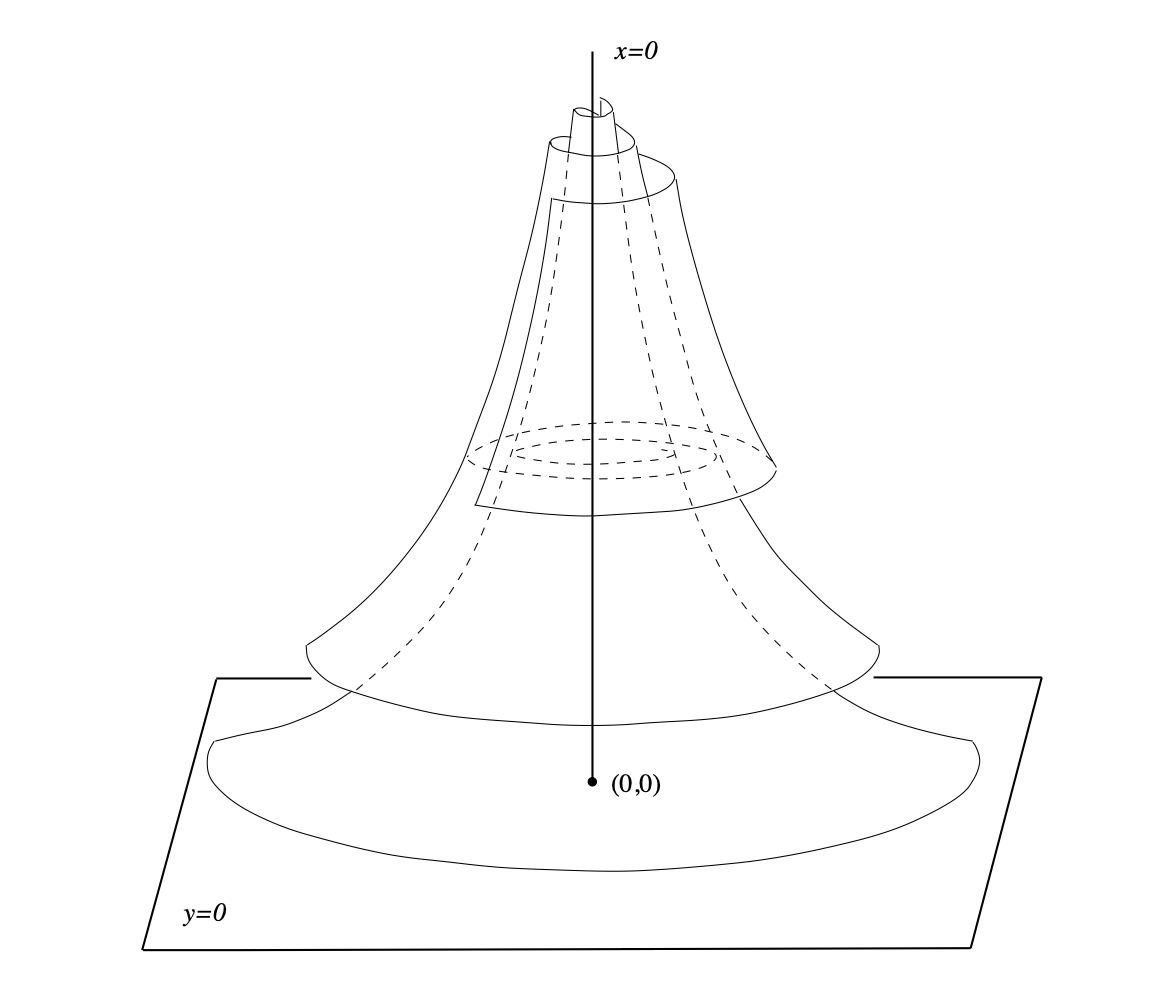# Generic planar algebraic vector fields are strongly minimal and disintegratedRepresentation of a complex hyberbolic singularity.

Abstract: In this article, we study model-theoretic properties of algebraic differential equations of order 2, defined over constant differential fields. In particular, we show that the set of solutions of a general differential equation of order 2 and of degree $$d≥3$$ in a differentially closed field is strongly minimal and disintegrated. We also give two other formulations of this result in terms of algebraic (non)-integrability and algebraic independence of the analytic solutions of a general planar algebraic vector field.

Categories:

Updated: